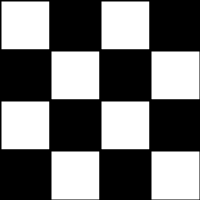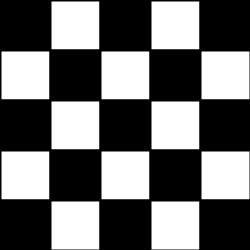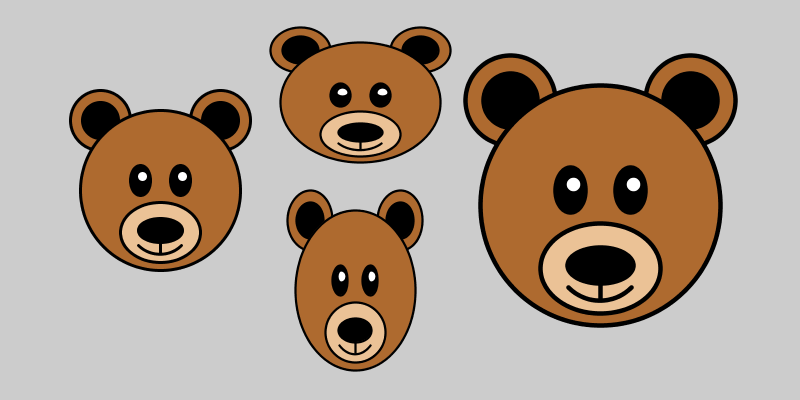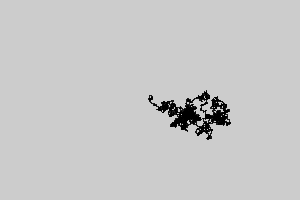## Kotlin & Processing basics

Start: 9/19/2019
Due: 10/2/2019, by the beginning of class
Collaboration: individual
Important Links: how to make your mac ready for MCS-178, how to make your Ubuntu machine ready for MCS-178, classpath-how-to, style guidelines, processing core library core.jar, readme.txt, gradesheet, zip how-to

### Overview

The goal of this assignment is to practice writing short Kotlin programs that

- use loops
- use conditional statements
- use the processing library functions
- process command line arguments

Before starting, follow instruction in the classpath how-to guide. I am assuming throughout this lab writeup that you have downloaded and saved the core.jar file in an appropriate place, and have defined your CLASSPATH environment variable appropriately, as described in the classpath how-to guide.

### The programs

1. Checkerboard. Write a program Checkerboard.kt that takes an integer command line argument n, and uses a nested loop construct to output an n-by-n checkerboard pattern like the ones below:

$java CheckerboardKt 4$ java CheckerboardKt 5The convention is that the lower left corner square is always black. Note that your program must work correctly for any positive integer n, not just for n equal to 4 or 5. Additionally, your program should check that the user provides exactly one command line argument (as a positive integer) before continuing; it should give error message and quit otherwise.

2. Bears. This exercise has two parts.

1. Write a Kotlin program Bear.kt that draws a cute cartoon face of a bear like the following.

$java BearKt2. By changing the arguments of all shape-drawing functions calls, turn your bear face drawing code into the function fun bear1(x: Float, y: Float, w: Float, h: Float) so that when bear1(x, y, w, h) is called, a bear face of width w, height h will be drawn with its upper left corner at (x, y). For example, this example run $ java BearsKtresults from the fact that the file Bears.kt contains the line

    size(800, 400)  

inside its settings() definition, and the lines

    bear1(60F,  80F, 200F, 200F)
bear1(260F, 20F, 200F, 150F)
bear1(280F, 180F, 150F, 200F)
bear1(450F,  40F, 300F, 300F)  

inside its draw() definition.

3. Now implement a function

fun bear2(x: Float, y: Float, w: Float, h: Float)

by changing the Bear.kt code so that calling bear2(x, y, w, h) works exactly like bear1(x, y, w, h). However, bear2 should use the translate and scale functions, and retains most of the bear face drawing code from Bear.kt.

3. Random walks. A drone begins flying aimlessly, starting at Pittman Hall. At each time step, the drone flies one meter in a random direction, either north, east, south, or west, with probability 25%. How far will the drone be from Pittman Hall after n steps? This process is known as a two-dimensional random walk. It is a discrete version of a natural phenomenon known as Brownian motion. It serves as a scientific model for an astonishing range of physical processes from the dispersion of ink flowing in water, to the formation of polymer chains in chemistry, to cascades of neurons firing in the brain.

1. Write a Kotlin program RandomWalker.kt that obtains an integer n from its command line argument and simulates the motion of a random walk for n steps. Draw the location point at each step (including the starting point), treating the starting point as the Cartesian Coordinates system origin (0, 0). Also, print the square of the final Euclidean distance from the origin. Here is an example run with n = 5000.

$java RandomWalkerKt 5000squared distance = 1250.0 Make sure the program checks the command line argument(s) and gives appropriate error message if not exactly one argument is given. 2. Write a program RandomWalkers.kt that obtains two integers n and trials from its command line arguments. In each of trials independent experiments, simulate a random walk of n steps and compute the squared distance (without using the processing library to display the points.). Output the mean squared distance (the average of the trials squared distances). Here are example runs of the program on various values of n and traials. $ kotlin RandomWalkersKt 100 10000      $kotlin RandomWalkersKt 400 2000 mean squared distance = 101.446 mean squared distance = 383.12$ kotlin RandomWalkersKt 100 10000      $kotlin RandomWalkersKt 800 5000 mean squared distance = 99.1674 mean squared distance = 811.8264$ kotlin RandomWalkersKt 200 1000       \$ kotlin RandomWalkersKt 1600 100000
mean squared distance = 195.75          mean squared distance = 1600.13064

As n increases, we expect the random walk to end up farther and farther away from the origin. But how much farther? Use RandomWalkersKt to formulate a hypothesis as to how the mean squared distance grows as a function of n. Use trials = 100,000 to get a sufficiently accurate estimate.

Make sure the program checks the command line argument(s) and gives appropriate error message if not exactly two arguments are given.

### Program style and format

Follow the guidelines in the Style Guidelines. Is your header complete? Did you comment appropriately? Is your code indented consistently? Did you use descriptive variable names? Did you follow the input and output specifications? Are there any redundant conditions?

You should create a “zip” archive file containing all Kotlin programs and your completed readme.txt file. Be sure to include the completed readme.txt file in your zip file. Read zip how-to if you do not know how to create a zip file. Ask your lab instructor for help if you need it.